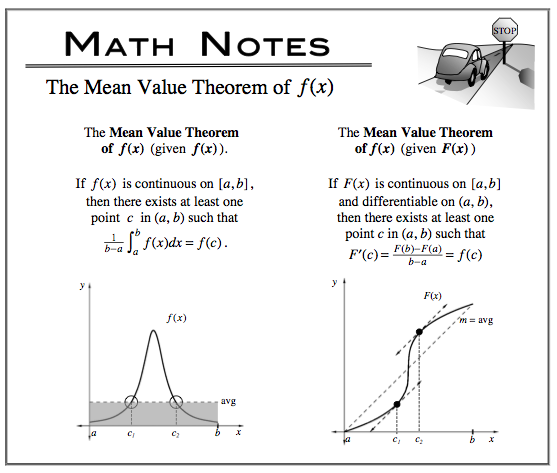### Home > CALC > Chapter 7 > Lesson 7.1.1 > Problem7-8

7-8.
1.For each function below, find its average value on the given integral and state the time t at which it equals its average value. Homework Help ✎

1. g(t) = 3t + 6 for [0, 8]

2. g(t) = 3et for [0, 1]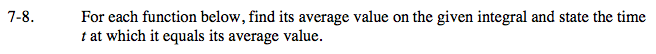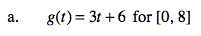Read the Math Note about how to compute the Mean Value of g(x), given g(x).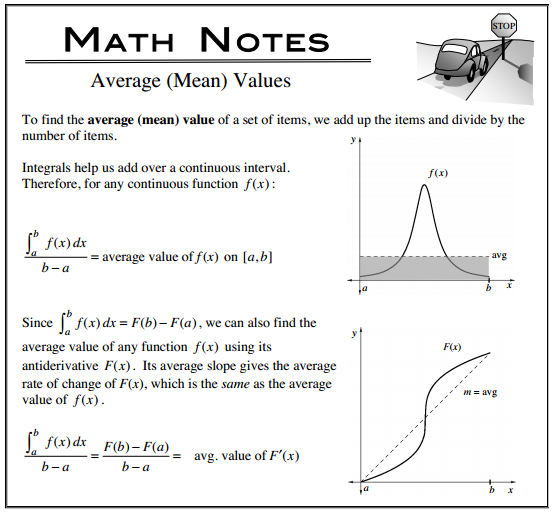To find the the time the function is at its average value, let g(t) = the average value and solve for t.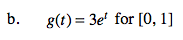Average Value = 3e − 3. Now find the time, t, that g(t) = its average value.

The Mean Value Theorem states that a differentiable function will reach its average (mean) value at least once on any closed interval.
Check your values of t in parts (a) and (b). Are they within the the given closed intervals?